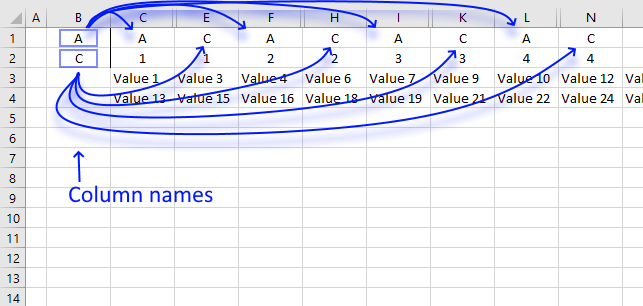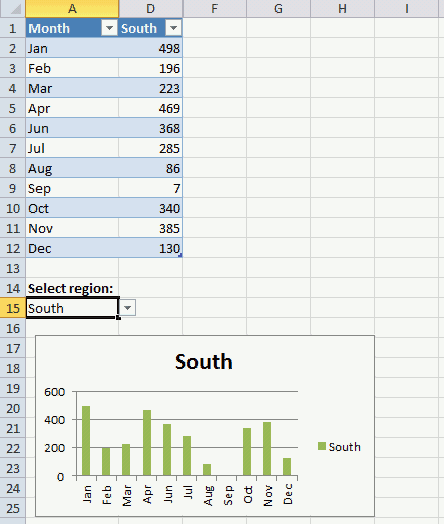Author: Oscar Cronquist Article last updated on October 25, 2019This article describes a macro that hides specific columns automatically based on values in two given cells.

I am also going to show you how to hide columns in an Excel Table programmatically. The Excel Table is the data source for a chart, it changes based on which value the user selects using a drop-down list.

Do you have a VBA to hide all columns of a range except columns whose header is found in specific cells?
eg:
B1 and B2 hold header names chosen by the user.
range C1 to P2 holds all headers.
Hide ALL columns except those sharing names found in B1 and B2?

### VBA code

```'Event code is rund when a cell changes
Private Sub Worksheet_Change(ByVal Target As Range)

'Dimension variables and declare data types
Dim Value As Range, Cells As Range, Cell As Variant
Dim c As Integer, b As Boolean

'Check if cell B1 or B2 is changed
If Not Intersect(Target, Range("B1:B2")) Is Nothing Then

'Stop screen from refreshing
Application.ScreenUpdating = False

'Unhide columns in cell range C:P
Worksheets("Cyril").Range("C1:P2").EntireColumn.Hidden = False

'Iterate through 0 (zero) to 13
For c = 0 To 13

'Save range object reference to variable Cells
Set Cells = Worksheets("Cyril").Range("C1:C2").Offset(0, c)

'Save False to variable b
b = False

'Iterate through each cell in variable Cells
For Each Cell In Cells

'Iterate through each value in cell range B1:B2 in worksheet Cyril
For Each Value In Worksheets("Cyril").Range("B1:B2")

'If statement checks whether variable Value equals variable Cell
If Value = Cell Then

'Save True to variable b
b = True
End If

'Continue with next value in variable Value
Next Value

'Continue with next cell
Next Cell

'Check if variable b is equal to False
If b = False Then

'Hide column
Cells.EntireColumn.Hidden = True
End If

'Continue with next number
Next c

'Show changes to Excel user
Application.ScreenUpdating = True
End If
End Sub
```

### Animated imageThe animated image above shows what happens when the user enters column header names in cell B1 and B2.

### Where to put the code?

1. Press with right mouse button on on the tab with sheet name "Cyril" located at the bottom of your screen.
2. Press with left mouse button on on "View Code", this opens the Visual Basic Editor and takes you to the worksheet module.
3. Paste VBA code to worksheet module.
Note, save your workbook with file extension *.xlsm (macro-enabled workbook) to attach the code.

### Hiding columns in a tableThe image above shows a drop-down list in cell A15 that allows you to control which data series you want to be graphed. When the value in cell A15 changes the following event macro hides all columns in the Excel Table except the chosen name. The chart changes as well and shows only what the Excel Table shows.

I highly recommend you use a slicer if you have Excel 2010 or a later version, there is no need for a macro.

```'Event code is rund when a cell is changed
Private Sub Worksheet_Change(ByVal Target As Range)

'Dimension variable and declare data type
Dim c As Integer

'Check if cell A15 is changed
If Not Intersect(Target, Range("A15")) Is Nothing Then

'Stop screen from refreshing
Application.ScreenUpdating = False

'Unhide all columns for table Table1
Range("Table1").EntireColumn.Hidden = False

'Iterate from 2 to the number of columns in Table1
For c = 2 To Range("Table1").Columns.Count

'Check if a cell is not equal to the value in cell A15
If Range("Table1[#All]").Cells(c) <> Range("A15") Then

'Hide column based on variable c representing the cell number in Table1
Range("Table1").Cells(c).EntireColumn.Hidden = True
End If

'Continue with next number in variable c
Next c

'Show changes to user
Application.ScreenUpdating = True

End If
End Sub

```

### Get the Excel fileHide-columns.xlsm